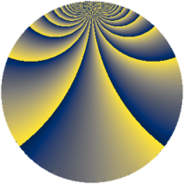# Properties

 Label 345.2.tLevel $345$ Weight $2$ Character orbit 345.t Rep. character $\chi_{345}(4,\cdot)$ Character field $\Q(\zeta_{22})$ Dimension $240$ Newform subspaces $1$ Sturm bound $96$ Trace bound $0$

# Learn more

## Defining parameters

 Level: $$N$$ $$=$$ $$345 = 3 \cdot 5 \cdot 23$$ Weight: $$k$$ $$=$$ $$2$$ Character orbit: $$[\chi]$$ $$=$$ 345.t (of order $$22$$ and degree $$10$$) Character conductor: $$\operatorname{cond}(\chi)$$ $$=$$ $$115$$ Character field: $$\Q(\zeta_{22})$$ Newform subspaces: $$1$$ Sturm bound: $$96$$ Trace bound: $$0$$

## Dimensions

The following table gives the dimensions of various subspaces of $$M_{2}(345, [\chi])$$.

Total New Old
Modular forms 520 240 280
Cusp forms 440 240 200
Eisenstein series 80 0 80

## Trace form

 $$240q + 28q^{4} - 4q^{5} - 4q^{6} + 24q^{9} + O(q^{10})$$ $$240q + 28q^{4} - 4q^{5} - 4q^{6} + 24q^{9} + 8q^{14} + 4q^{15} - 36q^{16} - 8q^{19} - 92q^{20} + 8q^{21} + 12q^{24} - 44q^{25} + 32q^{26} - 12q^{29} + 8q^{30} - 20q^{31} - 54q^{34} + 36q^{35} - 28q^{36} - 36q^{39} - 24q^{40} - 28q^{41} - 224q^{44} + 4q^{45} - 8q^{46} + 52q^{49} - 48q^{50} - 18q^{54} - 22q^{55} - 56q^{59} - 36q^{60} + 44q^{61} + 12q^{64} - 16q^{65} + 8q^{66} + 8q^{69} - 152q^{70} + 4q^{71} + 88q^{74} - 8q^{75} - 100q^{76} - 84q^{79} + 122q^{80} - 24q^{81} - 56q^{84} + 186q^{85} - 392q^{86} + 48q^{89} - 304q^{91} + 80q^{94} + 190q^{95} + 16q^{96} + 44q^{99} + O(q^{100})$$

## Decomposition of $$S_{2}^{\mathrm{new}}(345, [\chi])$$ into newform subspaces

Label Dim. $$A$$ Field CM Traces $q$-expansion
$$a_2$$ $$a_3$$ $$a_5$$ $$a_7$$
345.2.t.a $$240$$ $$2.755$$ None $$0$$ $$0$$ $$-4$$ $$0$$

## Decomposition of $$S_{2}^{\mathrm{old}}(345, [\chi])$$ into lower level spaces

$$S_{2}^{\mathrm{old}}(345, [\chi]) \cong$$ $$S_{2}^{\mathrm{new}}(115, [\chi])$$$$^{\oplus 2}$$